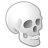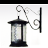# If - Then Statements in Field Calculator

8838
4
12-23-2013 08:45 AMNew Contributor II
I have a pretty simple set of replace functions I need to complete in an attribute table.  I have a feature class with approximately 135k records with a "MUN" attribute representing the four digit code of the applicable municipality.  I have a new field called "MUNI" in which I wish to populate the correct Municipal name.  There are 12 municipalities.

I've worked on several variations of the code in field calculator but none seem to work; general syntax error.  Coding and languages were never my strong suit so it doesn't necessarily surprise me but nevertheless.  I had a working expression to do just this but it had been deleted.

If [MUN] = 0901 Then
[MUNI] = Bayonne
ELSEIF [MUN] = 0902 Then
[MUNI] = East Newark
ELSEIF [MUN] = 0903 Then
[MUNI] = Guttenberg
ELSEIF [MUN] = 0904 Then
[MUNI] = Harrison
ELSEFIF [MUN] = 0905 Then
[MUNI] = Hoboken
ELSEIF [MUN] = 0906 Then
[MUNI] = Jersey City
ELSEIF [MUN] = 0907 Then
[MUNI] = Kearny
ELSEIF [MUN]= 0908 Then
[MUNI] = North Bergen
ELSEIF [MUN] = 0909 Then
[MUNI] = Secaucus
ELSEIF [MUN] = 0910 Then
[MUNI] = Union City
ELSEIF [MUN] = 0911 Then
[MUNI] = Weehawken
ELSEIF [MUN] = 0912 Then
[MUNI] = West New York

A simple expression I should be able to build with little effort but not having done this for a bit is hanging me up!
Tags (2)
4 RepliesNew Contributor II
Other variation.  Note all fields are string.

P-L SC:

If [MUN] = "0901" Then
output = "Bayonne"
ELSEIF [MUN] = "0902" Then
output = "East Newark"
ELSEIF [MUN] = "0903" Then
output = "Guttenberg"
ELSEIF [MUN] = "0904" Then
output = "Harrison"
ELSEFIF [MUN] = "0905" Then
output = "Hoboken"
ELSEIF [MUN] = "0906" Then
output = "Jersey City"
ELSEIF [MUN] = "0907" Then
output = "Kearny"
ELSEIF [MUN]= "0908" Then
output = "North Bergen"
ELSEIF [MUN] = "0909" Then
output = "Secaucus"
ELSEIF [MUN] = "0910" Then
output = "Union City"
ELSEIF [MUN] = "0911" Then
output = "Weehawken"
ELSEIF [MUN] = "0912" Then
output = "West New York"

MUNI=outputRegular Contributor
Hello Jason,

I'd use the python editor in Field editor. Then use "Show Codebook" and define a method (see screenshot). Your Pre-Logic Script Code should look something like this:
```def customReplace(x):
if x=='0901':
return 'Bayonne'
elif x=='0902':
return 'East Newark'
elif x=='0903':
return 'Guttenberg'
#etc.
else:

Then call the method from the field calculator. Where "MUNI = "
`customReplace( !MUN! )`

That should work :)Frequent Contributor III
Your making this way to hard.

if you have a the muni code list simply add the table to an ArcGIS Session.  Join the feature to the table muni table via the 4 digit code.  then populate your name field with the name field from the muni table.

Total time to set this up should be less than 2 minutes.  Then depending on your system configuration a little more time to perform the calculation.

If it is a recurring operation MS Access is quick and easy as well.  Have your 4 digit code table in there as a LUT.

Create a query that joins the 2 tables and perform the update with the double click of a query.byMVP Honored Contributor
Jason's code comes the closest for VB, but you have to close all of the conditions with an End If statement at the end of the code block.

I would use the Select Case syntax instead, since ELSEIF may fail after 7 levels (based on past experience with VBA).  My Code would be:

Parser: VB Script

Use Code Block: Checked

Pre-Logic Code Block:
```Select Case [MUN]
Case "0901" output = "Bayonne"
Case "0902" output = "East Newark"
Case "0903" output = "Guttenberg"
Case "0904" output = "Harrison"
Case "0905" output = "Hoboken"
Case "0906" output = "Jersey City"
Case "0907" output = "Kearny"
Case "0908" output = "North Bergen"
Case "0909" output = "Secaucus"
Case "0910" output = "Union City"
Case "0911" output = "Weehawken"
Case "0912" output = "West New York"
Case Else    output = [MUNI]
End Select```

Expression (MUNI field)
output